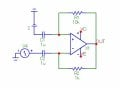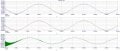# Positive or negative feedback op amp?

#### kubeek

Joined Sep 20, 2005
5,754
I think that circuit is called a howland current source, and i'd say both positive and negative feedback are working in it.

#### OBW0549

Joined Mar 2, 2015
3,566
Can someone explain to me, how to determine which feedback is active in circuit? As I can see from simulation, in this case negative feedback is active because op amp works in linear region. Is there any way to determine that with some hand-calculation?
For this circuit, the determination is simple: the feedback from the op amp output back to its (+) input is attenuated by half (R1/(R1+R2)), while the feedback to the (-) input is reduced to 1/4 (R3/(R3/R4)) of the voltage at the output. Therefore, the positive feedback dominates. Be careful about assuming the op amp is working in its linear region: it appears to be saturated against the negative supply rail.

I think that circuit is called a howland current source, and i'd say both positive and negative feedback are working in it.
The mere fact that the circuit contains both positive and negative feedback paths DOES NOT make it a Howland current source.

#### kubeek

Joined Sep 20, 2005
5,754
The mere fact that the circuit contains both positive and negative feedback paths DOES NOT make it a Howland current source.
You are right master Obi Wan, I judged too soon#### Nemanja_95

Joined Nov 14, 2017
24
For this circuit, the determination is simple: the feedback from the op amp output back to its (+) input is attenuated by half (R1/(R1+R2)), while the feedback to the (-) input is reduced to 1/4 (R3/(R3/R4)) of the voltage at the output. Therefore, the positive feedback dominates. Be careful about assuming the op amp is working in its linear region: it appears to be saturated against the negative supply rail.
Ok, does it mean that when I analyze which feedback dominates (in general), first I remove input signals and then watch what is happening with (+) and (-) inputs of op amp?

#### OBW0549

Joined Mar 2, 2015
3,566
For purposes of identifying which feedback path dominates, the input sources can be set to zero since their specific values (7 volts and 1 volt, in this case) are irrelevant. What matters are the feedback transfer functions (or, more simply, the voltage feedback ratios) between the output and the (+) input, and from the output to the (-) input. Whichever is greater, that one dominates.

#### Nemanja_95

Joined Nov 14, 2017
24
It's clear now.
Thank you very much, it was very helpful!

•OBW0549

#### AnalogKid

Joined Aug 1, 2013
9,283
Be careful about assuming the op amp is working in its linear region: it appears to be saturated against the negative supply rail.
Disagree. The voltages all work out for battery and resistor values shown. Plus, a saturated output would be closer to the -15 V rail; 4 V headroom seems large.
The mere fact that the circuit contains both positive and negative feedback paths DOES NOT make it a Howland current source.
Agree. The Howland pump requires equal impedance ratios.

ak

•OBW0549

#### OBW0549

Joined Mar 2, 2015
3,566
Disagree. The voltages all work out for battery and resistor values shown. Plus, a saturated output would be closer to the -15 V rail; 4 V headroom seems large.
Thinking about it, I realize you're right: the op amp very likely is operating in its linear region (unless by some magic its negative saturation level were precisely -11V), because the two inputs are at equal voltages.

However, even if it's operating in its linear region, that operating point is not stable: if the op amp is not saturated now, it certainly will be in short order since any deviation of the output voltage will change the differential input voltage in such a way as to amplify that deviation. In other words, as I suggested in post #6 above, the net feedback is positive.

#### AnalogKid

Joined Aug 1, 2013
9,283
As an unbalanced Howland pump, it has a high-but-not-infinite output impedance. RTS used a version of this to create a multi-amp power supply with a synthesized 200 ohm output impedance.

ak

#### Nemanja_95

Joined Nov 14, 2017
24
I have to go back to this, to make sure that I understand the principle. So, now I have an circuit with two capacitors instead of resistor...

1. If OP-amp output rises C2 is charging faster than C1, so output fails. Then, if output falls C2 is discharging faster than C1 so output rises again --> Negative feedback
2. Also, if I analize this like previus example, I would have V- > V+ --> Negative feedback.
(If R2 > R1 then this would be positive feedback)

I don't think this circuit is doing anything smart, I just want to know is this analyzing (1 and 2) correct?#### AnalogKid

Joined Aug 1, 2013
9,283
There is no DC reference potential for either opamp input, so the output will change due to input offset voltage error until it saturates.

ak